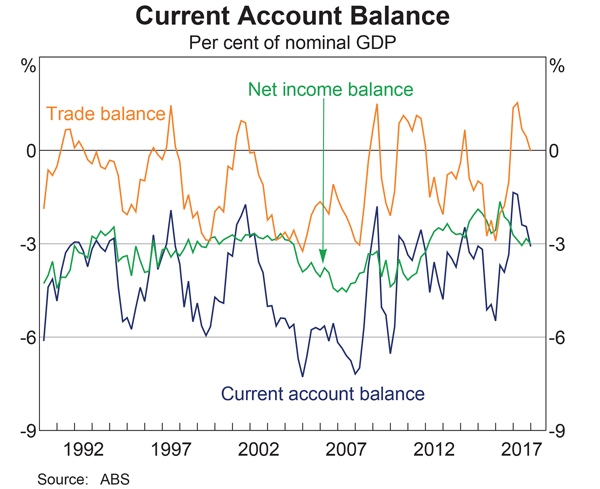# Fight Finance

#### CoursesTagsRandomAllRecentScores

For a price of $10.20 each, Renee will sell you 100 shares. Each share is expected to pay dividends in perpetuity, growing at a rate of 5% pa. The next dividend is one year away (t=1) and is expected to be$1 per share.

The required return of the stock is 15% pa.

Would you like to the shares or politely ?

 Portfolio Details Stock Expected return Standard deviation Correlation Dollars invested A 0.1 0.4 0.5 60 B 0.2 0.6 140

What is the expected return of the above portfolio?

The following cash flows are expected:

• 10 yearly payments of $60, with the first payment in 3 years from now (first payment at t=3 and last at t=12). • 1 payment of$400 in 5 years and 6 months (t=5.5) from now.

What is the NPV of the cash flows if the discount rate is 10% given as an effective annual rate?

Acquirer firm plans to launch a takeover of Target firm. The deal is expected to create a present value of synergies totaling $105 million. A scrip offer will be made that pays the fair price for the target's shares plus 75% of the total synergy value.  Firms Involved in the Takeover Acquirer Target Assets ($m) 6,000 700 Debt ($m) 4,800 400 Share price ($) 40 20 Number of shares (m) 30 15

Ignore transaction costs and fees. Assume that the firms' debt and equity are fairly priced, and that each firms' debts' risk, yield and values remain constant. The acquisition is planned to occur immediately, so ignore the time value of money.

Calculate the merged firm's share price and total number of shares after the takeover has been completed.

A mining firm has just discovered a new mine. So far the news has been kept a secret.

The net present value of digging the mine and selling the minerals is $250 million, but$500 million of new equity and $300 million of new bonds will need to be issued to fund the project and buy the necessary plant and equipment. The firm will release the news of the discovery and equity and bond raising to shareholders simultaneously in the same announcement. The shares and bonds will be issued shortly after. Once the announcement is made and the new shares and bonds are issued, what is the expected increase in the value of the firm's assets $(\Delta V)$, market capitalisation of debt $(\Delta D)$ and market cap of equity $(\Delta E)$? Assume that markets are semi-strong form efficient. The triangle symbol $\Delta$ is the Greek letter capital delta which means change or increase in mathematics. Ignore the benefit of interest tax shields from having more debt. Remember: $\Delta V = \Delta D+ \Delta E$ Calculate the price of a newly issued ten year bond with a face value of$100, a yield of 8% pa and a fixed coupon rate of 6% pa, paid semi-annually. So there are two coupons per year, paid in arrears every six months.

Many Australian home loans that are interest-only actually require payments to be made on a fully amortising basis after a number of years.

You decide to borrow $600,000 from the bank at an interest rate of 4.25% pa for 25 years. The payments will be interest-only for the first 10 years (t=0 to 10 years), then they will have to be paid on a fully amortising basis for the last 15 years (t=10 to 25 years). Assuming that interest rates will remain constant, what will be your monthly payments over the first 10 years from now, and then the next 15 years after that? The answer options are given in the same order. Mr Blue, Miss Red and Mrs Green are people with different utility functions. Each person has$50 of initial wealth. A coin toss game is offered to each person at a casino where the player can win or lose $50. Each player can flip a coin and if they flip heads, they receive$50. If they flip tails then they will lose $50. Which of the following statements is NOT correct?You bought a 1.5 year (18 month) futures contract on oil. Oil storage costs are 4% pa continuously compounded and oil pays no dividends. The futures contract is entered into when the oil price is$40 per barrel and the risk-free rate of interest is 10% per annum with continuous compounding.

Which of the following statements is NOT correct?

This question is about the Balance of Payments. Australia's current account as a percent of nominal gross domestic product (GDP) per annum is shown in the graph below.Assume that all foreign and domestic assets are either debt which makes interest income or equity which makes dividend income, and vice versa for liabilities which cost interest and dividend payments, respectively.

Which of the following statements is NOT correct?